# How to calculate age in Excel: from date of birth, between two dates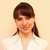by , updated on

The tutorial shows different ways to get age from birthday in Excel. You will learn a handful of formulas to calculate age as a number of complete years, get exact age in years, months and days at today's date or a particular date.

There is no special function to calculate age in Excel, however there exist a few different ways to convert date of birth to age. This tutorial will explain the advantages and drawbacks of each way, shows how to make a perfect age calculation formula in Excel and tweak it for solving some specific tasks.

## How to calculate age from date of birth in Excel

In everyday life, the question "How old are you?" usually implies an answer indicating how many years you have been alive. In Microsoft Excel, you can make a formula to compute an exact age in months, days, hours and even minutes. But let's be traditional, and learn how to calculate age from DOB in years first.

### Basic Excel formula for age in years

How do you normally figure out someone's age? Simply by subtracting the birth date from the current date. This conventional age formula can also be used in Excel.

Assuming a birthdate is in cell B2, the formula to calculate age in years goes as follows:

`=(TODAY()-B2)/365`

The first part of the formula (TODAY()-B2) returns the difference between the current date and date of birth is days, and then you divide that number by 365 to get the numbers of years.

The formula is obvious and easy-to-remember, however, there is a tiny problem. In most cases, it returns a decimal number as demonstrated in the screenshot below.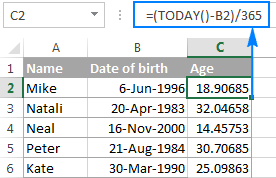To display the number of complete years, use the INT function to round a decimal down to the nearest integer:

`=INT((TODAY()-B2)/365)`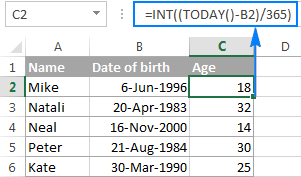Drawbacks: Using this age formula in Excel produces pretty accurate results, but not flawless. Dividing by the average number of days in a year works fine most of the time, but sometimes it gets the age wrong. For example, if someone was born on February 29 and today is February 28, the formula will make a person one day older.

As an alternative, you can divide by 365.25 instead of 365 since every fourth year has 366 days. However, this approach is not perfect either. For example, if you are calculating the age of a child who hasn't yet lived through a leap year, dividing by 365.25 produces a wrong result.

Overall, subtracting the birth date from the current date works great in normal life, but is not the ideal approach in Excel. Further on in this tutorial, you will learn a couple of special functions that calculate age faultlessly regardless of the year.

### Calculate age from date of birth with YEARFRAC function

A more reliable way to convert DOB to age in Excel is using the YEARFRAC function that returns the fraction of the year, i.e. the number of whole days between two dates.

The syntax of the YEARFRAC function is as follows:

YEARFRAC(start_date, end_date, [basis])

The first two arguments are obvious and hardly require any additional explanation. Basis is an optional argument that defines the day count basis to use.

To make a perfectly true age formula, supply the following values to the YEARFRAC function:

• Start_date - date of birth.
• End_date - TODAY() function to return the today's date.
• Basis - use basis 1 that tells Excel to divide the actual number of days per month by the actual number of days per year.

Considering the above, an Excel formula to calculate age from date of birth is as follows:

YEARFRAC(date of birth, TODAY(), 1)

Assuming the birthdate is in cell B2, the formula takes the following shape:

`=YEARFRAC(B2, TODAY(), 1)`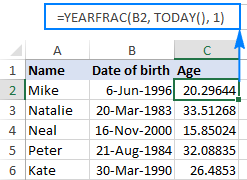Like in the previous example, the result of the YEARFRAC function is also a decimal number. To fix this, use the ROUNDDOWN function with 0 in the last argument because you don't want any decimal places.

So, here's an improved YEARFRAC formula to calculate age in Excel:

`=ROUNDDOWN(YEARFRAC(B2, TODAY(), 1), 0)`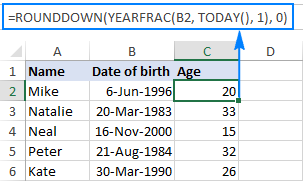### Calculate age in Excel with DATEDIF

One more way to convert date of birth to age in Excel is using the DATEDIF function:

DATEDIF(start_date, end_date, unit)

This function can return the difference between two dates in various time units such as years, months and days, depending on the value you supply in the unit argument:

• Y - returns the number of complete years between the start and end dates.
• M - returns the number of complete months between the dates.
• D - returns the number of days between the two dates.
• YM - returns months, ignoring days and years.
• MD - returns the difference in days, ignoring months and years.
• YD - returns the difference in days, ignoring years.

Since we aim to calculate age in years, we are using the "y" unit:

DATEDIF(date of birth, TODAY(), "y")

In this example, the DOB is in cell B2, and you reference this cell in your age formula:

`=DATEDIF(B2, TODAY(), "y")`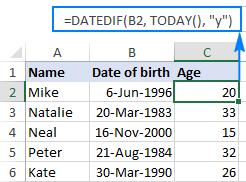No additional rounding function is needed in this case because a DATEDIF formula with the "y" unit calculates the number of full years:## How to get age from birthday in years, months and days

As you have just seen, calculating age as the number of full years that the person has lived is easy, but it is not always sufficient. If you want to know the exact age, i.e. how many years, months and days there are between someone's birth date and the current date, write 3 different DATEDIF functions:

1. To get the number of years: `=DATEDIF(B2, TODAY(), "Y")`
2. To get the number of months: `=DATEDIF(B2, TODAY(), "YM")`
3. To get the number of days: `=DATEDIF(B2,TODAY(),"MD")`

Where B2 is the date of birth.

And then, concatenate the above functions in a single formula, like this:

`=DATEDIF(B2,TODAY(),"Y") & DATEDIF(B2,TODAY(),"YM") & DATEDIF(B2,TODAY(),"MD")`

The above formula returns 3 numbers (years, months, and days) concatenated in a single text string, as shown in the screenshot below: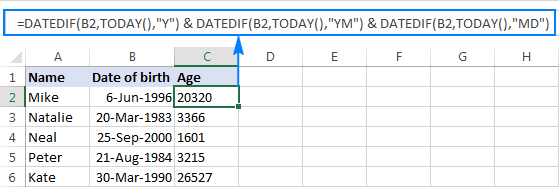Does not make much sense, uh? To make the results more meaningful, separate the numbers with commas and define what each value means:

`=DATEDIF(B2,TODAY(),"Y") & " Years, " & DATEDIF(B2,TODAY(),"YM") & " Months, " & DATEDIF(B2,TODAY(),"MD") & " Days"`

The result looks much better now: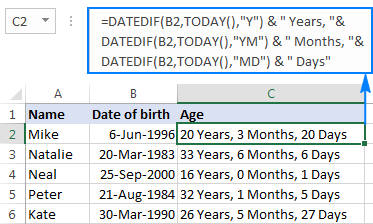The formula works great, but you can improve it even further by hiding zero values. For this, add 3 IF statements that check for 0's, one per each DATEDIF:

`=IF(DATEDIF(B2, TODAY(),"y")=0,"",DATEDIF(B2, TODAY(),"y")&" years, ")& IF(DATEDIF(B2, TODAY(),"ym")=0,"",DATEDIF(B2, TODAY(),"ym")&" months, ")& IF(DATEDIF(B2, TODAY(),"md")=0,"",DATEDIF(B2, TODAY(),"md")&" days")`

The following screenshot demonstrates the final Excel age formula in action - it returns age in years, months, and days, displaying only non-zero values: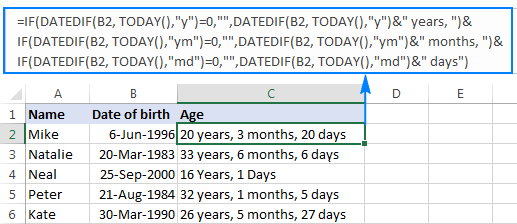Tip. If you are looking for an Excel formula to calculate age in years and months, take the above formula and remove the last IF(DATEDIF()) block that computes days.

## Specific formulas to calculate age in Excel

The generic age calculation formulas discussed above work great in most cases. In some situations, however, you may need something very specific. Of course, it's not possible to cover every and each scenario, but the following examples will give you some ideas on how you can tweak an age formula depending on your particular task.

### How to calculate age on a specific date in Excel

If you want to know someone's age at a certain date, use the DATEDIF age formula discussed above, but replace the TODAY() function in the 2nd argument with the specific date.

Assuming the birth date is in B1, the following formula will return age as of 1 January 2020:

`=DATEDIF(B1, "1/1/2020","Y") & " Years, " & DATEDIF(B1, "1/1/2020","YM") & " Months, " & DATEDIF(B1, "1/1/2020", "MD") & " Days"`

To make your age formula more flexible, you can input the date in some cell and reference that cell in your formula:

`=DATEDIF(B1, B2,"Y") & " Years, "& DATEDIF(B1,B2,"YM") & " Months, "&DATEDIF(B1,B2, "MD") & " Days"`

Where B1 is the DOB, and B2 is the date on which you want to calculate age.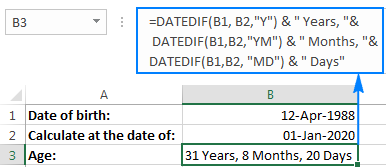### Calculate age in a certain year

This formula comes in handy in situations when the complete date to calculate at is not defined, and you know only the year.

Let's say you are working with a medical database, and your goal is to find out the patients' age at the time they underwent the last full medical examination.

Assuming the dates of birth are in column B beginning with row 3, and the year of the last medical examination is in column C, the age calculation formula goes as follows:

`=DATEDIF(B3,DATE(C3, 1, 1),"y")`

Because the exact date of the medical examination is not defined, you use the DATE function with an arbitrary date and month argument, e.g. DATE(C3, 1, 1).

The DATE function extracts the year from cell B3, makes a complete date using the month and day numbers that you supplied (1-Jan in this example), and passes that date to DATEDIF. As the result, you get the patient's age as of January 1 of a particular year: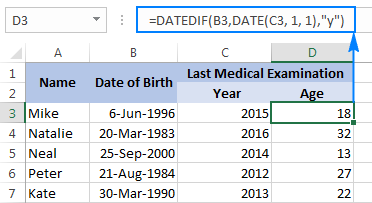### Find out a date when a person attains N years of age

Supposing your friend was born on 8 March 1978. How do you know on what date he completes his 50 years of age? Usually, you'd simply add 50 years to the person's birthdate. In Excel, you do the same using the DATE function:

`=DATE(YEAR(B2) + 50, MONTH(B2), DAY(B2))`

Where B2 is the date of birth.

Instead of hard-coding the number of years in the formula, you can reference a certain cell where your users can input any number of years (F1 in the screenshot below):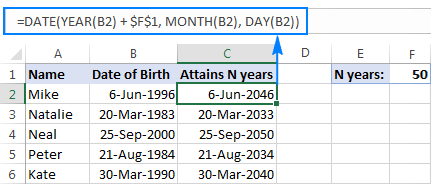### Calculate age from day, month and year in different cells

When a birthdate is split into 3 different cells (e.g. year is in B3, month in C3 and day in D3), you can calculate age in this way:

• Get the date of birth by using the DATE and DATEVALUE functions:
`DATE(B3,MONTH(DATEVALUE(C3&"1")),D3)`
• Embed the above formula into DATEDIF to calculate age from date of birth in years, months, and days:`=DATEDIF(DATE(B3, MONTH(DATEVALUE(C3&"1")), D3), TODAY(), "y") & " Years, "& DATEDIF(DATE(B3, MONTH(DATEVALUE(C3&"1")), D3),TODAY(), "ym") & " Months, "& DATEDIF(DATE(B3, MONTH(DATEVALUE(C3&"1")), D3), TODAY(), "md") & " Days"`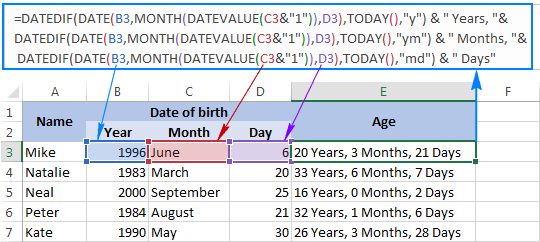For more examples of calculating the number of days before/after a date, please see How to calculate days since or until date in Excel.

## Age calculator in Excel

If you'd like to have your own age calculator in Excel, you can make one by using a few different DATEDIF formulas explained below. If you'd rather not reinvent the wheel, you can use the age calculator created by our Excel professionals.

### How to create an age calculator in Excel

Now that you know how to make an age formula in Excel, you can build a custom age calculator, for example this one:

What you see above is an embedded Excel Online sheet, so feel free to enter your birthdate in the corresponding cell, and you will get your age in a moment.

The calculator uses the following formulas to compute age based on the date of birth in cell A3 and today's date.

• Formula in B5 calculates age in years, months, and days:`=DATEDIF(B2,TODAY(),"Y") & " Years, " & DATEDIF(B2,TODAY(),"YM") & " Months, " & DATEDIF(B2,TODAY(),"MD") & " Days"`
• Formula in B6 calculates age in months:`=DATEDIF(\$B\$3,TODAY(),"m")`
• Formula in B7 calculates age in days:`=DATEDIF(\$B\$3,TODAY(),"d")`

If you have some experience with Excel Form controls, you can add an option to compute age at a specific date, like shown in the following screenshot: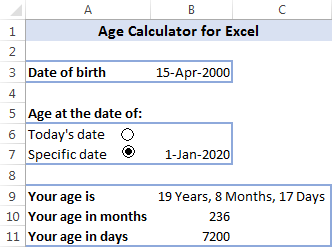For this, add a couple of option buttons (Developer tab > Insert > Form controls > Option Button), and link them to some cell. And then, write an IF/DATEDIF formula to get age either at today's date or at the date specified by the user.

The formula works with the following logic:

• If the Today's date option box is selected, value 1 appears in the linked cell (I5 in this example), and the age formula calculates based on the today date:`IF(\$I\$5=1, DATEDIF(\$B\$3,TODAY(),"Y") & " Years, " & DATEDIF(\$B\$3,TODAY(), "YM") & " Months, " & DATEDIF(\$B\$3, TODAY(), "MD") & " Days")`
• If the Specific date option button is selected AND a date is entered in cell B7, age is calculated at the specified date:`IF(ISNUMBER(\$B\$7), DATEDIF(\$B\$3, \$B\$7,"Y") & " Years, " & DATEDIF(\$B\$3, \$B\$7,"YM") & " Months, " & DATEDIF(\$B\$3, \$B\$7,"MD") & " Days", ""))`

Finally, nest the above functions into each other, and you will get the complete age calculation formula (in B9):

`=IF(\$I\$5=1, DATEDIF(\$B\$3, TODAY(), "Y") & " Years, " & DATEDIF(\$B\$3, TODAY(), "YM") & " Months, " & DATEDIF(\$B\$3, TODAY(), "MD") & " Days", IF(ISNUMBER(\$B\$7), DATEDIF(\$B\$3, \$B\$7,"Y") & " Years, " & DATEDIF(\$B\$3, \$B\$7,"YM") & " Months, " & DATEDIF(\$B\$3, \$B\$7,"MD") & " Days", ""))`

The formulas in B10 and B11 work with the same logic. Of course, they are much simpler because they include just one DATEDIF function to return age as the number of complete months or days, respectively.

To learn the details, I invite you to download this Excel Age Calculator and investigate the formulas in cells B9:B11.

### Ready-to-use age calculator for Excel

The users of our Ultimate Suite don't have to bother about making their own age calculator in Excel - it is only a couple of clicks away:

1. Select a cell where you want to insert an age formula, go to the Ablebits Tools tab > Date & Time group, and click the Date & Time Wizard button.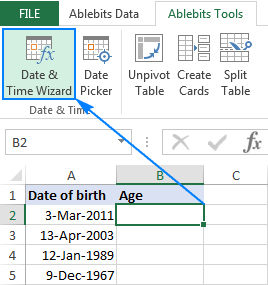2. The Date & Time Wizard will start, and you go directly to the Age tab.
3. On the Age tab, there are 3 things for you to specify:
• Data of birth as a cell reference or a date in the mm/dd/yyyy format.
• Age at today's date or specific date.
• Choose whether to calculate age in days, months, years, or exact age.
4. Click the Insert formula button.

Done!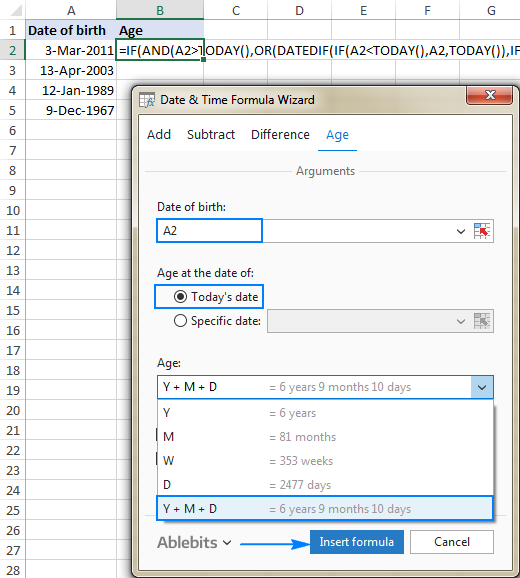The formula is inserted in the selected cell momentarily, and you double-click the fill handle to copy it down the column.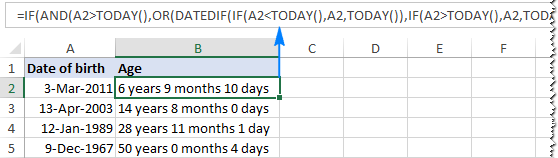As you may have noticed, the formula created by our Excel age calculator is more complex than the ones we've discussed so far, but it caters for singular and plural of time units such as "day" and "days".

If you'd like to get rid of zero units like "0 days", select the Do not show zero units check box: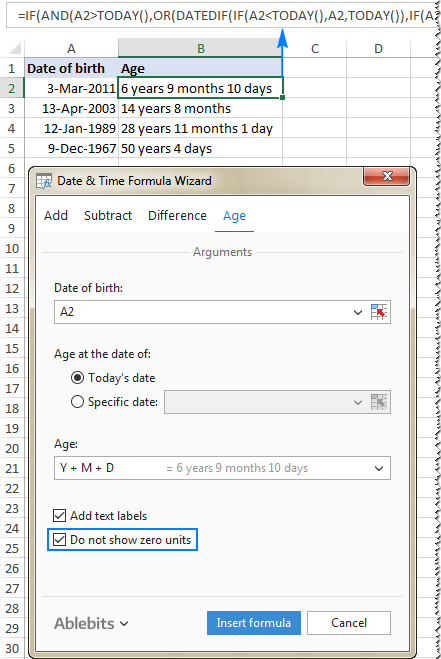If you are curious to test this age calculator as well as to discover 60 more time-saving add-ins for Excel, you are welcome to download a trial version of our Ultimate Suite at the end of this post.

## How to highlight certain ages (under or over a specific age)

In some situations, you may need not only calculate age in Excel, but also highlight cells which contain ages that are under or over a particular age.

If your age calculation formula returns the number of complete years, then you can create a regular conditional formatting rule based on a simple formula like these ones:

• To highlight ages equal to or greater than 18: =\$C2>=18
• To highlight ages under 18: =\$C2<18

Where C2 is the top-most cell in the Age column (not including the column header).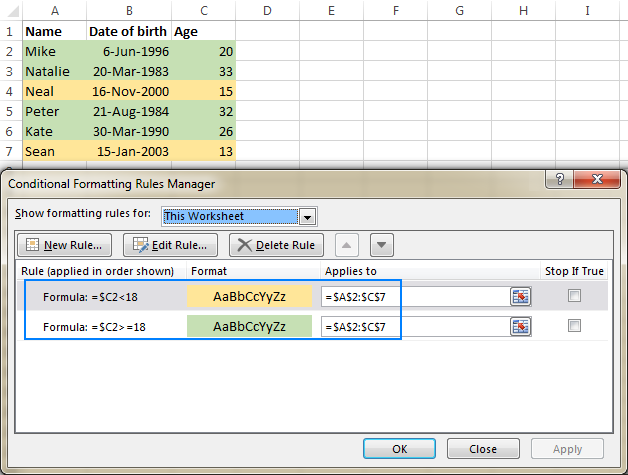But what if your formula displays age in years and months, or in years, months and days? In this case, you will have to create a rule based on a DATEDIF formula that calculates age from date of birth in years.

Supposing the birthdates are in column B beginning with row 2, the formulas are as follows:

• To highlight ages under 18 (yellow):`=DATEDIF(\$B2, TODAY(),"Y")<18`
• To highlight ages between 18 and 65 (green):`=AND(DATEDIF(\$B2, TODAY(),"Y")>=18, DATEDIF(\$B2, TODAY(),"Y")<=65)`
• To highlight ages over 65 (blue):`=DATEDIF(\$B2, TODAY(),"Y")>65`

To create rules based on the above formulas, select the cells or entire rows that you want to highlight, go to the Home tab > Styles group, and click Conditional Formatting > New Rule… > Use a formula to determine which cells to format.

The detailed steps can be found here: How to make a conditional formatting rule based on formula.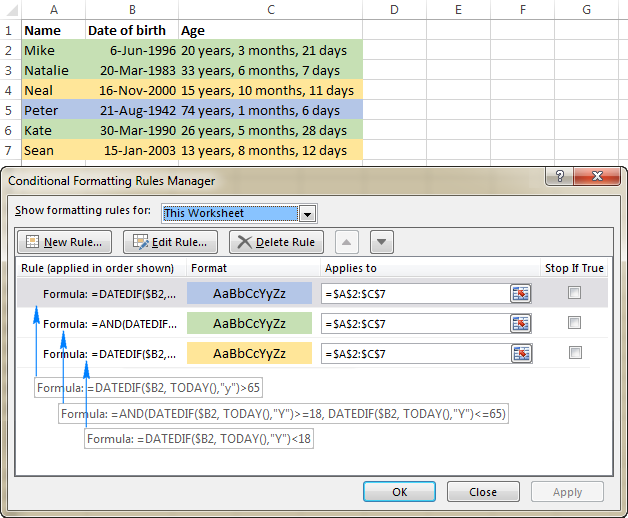This is how you calculate age in Excel. I hope the formulas were easy for you to learn and you will give them a try in your worksheets. Thank you for reading and hope to see you on our blog next week!